# MCQs on Electric Circuits

##### Page 24 of 63. Go to page 1 2 3 4 5 6 7 8 9 10 11 12 13 14 15 16 17 18 19 20 21 22 23 24 25 26 27 28 29 30 31 32 33 34 35 36 37 38 39 40 41 42 43 44 45 46 47 48 49 50 51 52 53 54 55 56 57 58 59 60 61 62 63
01․ Which of the following has positive temperature coefficient ?
Germanium.
Gold.
Paper.
Rubber.

Normally metallic substance has positive temperature coefficient. Gold is a metallic substance.

02․ Two resistances R1 and R2 give combined resistances 4.5 Ω and 1 Ω when they are connected in series and parallel respectively. What would be the values of these resistances?
3 Ω and 6 Ω.
1.5 Ω and 3 Ω.
3 Ω and 9 Ω.
6 Ω and 9 Ω.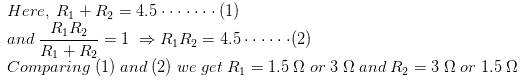03․ Which of the following may be value of resistivity of copper?
1.7 X 10 - 6.
1.7 X 10 - 5.
1.7 X 10 - 4.
1.7 X 10 - 3.

The value of resistivity of copper is 1.7 X 10 - 6.

04․ Two equal resistors R connected in series across a voltage source V dissipate power P. What would be the power dissipated in the same resistors when they are connected in parallel across the same voltage source?
4P.
P.
2P.
16P.

Say R is the resistance of the identical two resistors. When they are connected in series across a voltage source V, the equivalent resistance of the combination is 2R and then total power dissipated by the resistors will be P = V2 / 2R. When they are connected in parallel across the same voltage source V, the equivalent resistance of the combination is R/2 then total power dissipated by the resistors will be V2 / ( R / 2) = 4V2 / 2R = 4P.

05․ Two identical resistors are first connected in parallel then in series. The ratio of resultant resistance of the first combination to the second will be
4.
0.25.
2.
0.5.

Let us consider the value resistance of the equal resistors is R. So equivalent resistance of parallel combination of the resistors is R / 2, and equivalent resistance of series combination of the resistors is 2R. So ratio of these two combination will be (R / 2) / 2R = 1 / 4 = 0.25.

06․ The ratio of the resistance of a 200 W, 230 V lamp to that of a 100 W, 115 V lamp will be
0.5.
2.
4.
0.25.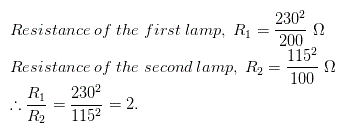07․ The resistance of 200 W, 200 V lamp is
100 Ω.
200 Ω.
400 Ω.
800 Ω.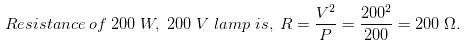.

08․ Two 1 kΩ, 1 W resistors are connected in series. Their combine resistance and wattage will be
2 kΩ, 0.5 W.
1 kΩ, 1 W.
0.5 kΩ, 2 W.
2 kΩ, 1 W.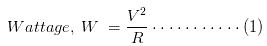Here, for each resistor, W = 1 W and R = 1 kΩ and putting these values in equation (1), we get V2 = 1, When two 1 kΩ resistance are connected in series, combined resistance will be 2 kΩ and putting this value and and V2 = 1 in equation (1) we again get, combined wattage W = 1 / 2 watt.

09․ Three 3Ω resistors are connected to form a triangle. What is the resistance between any two of the corner?
9 Ω.
6 Ω.
3 Ω.
2 Ω.

Whenever we look at the said triangle from any two of its corners, we will find that it is just a parallel combination of one 3 Ω and one 6 Ω (3 + 3 = 6) resistor. Thus, the resistance across these two corner points of the triangle will be (3 × 6)/ (3 + 6) = 18 / 9 = 2 Ω.

10․ A wire of 0.14 mm diameter and specific resistance 9.6 Ω - cm is 440 cm long. The resistance of the wire will be
9.6 Ω.
11.3 Ω.
13.7 Ω.
27.4 Ω.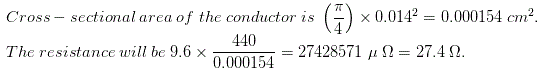<<<2223242526>>>